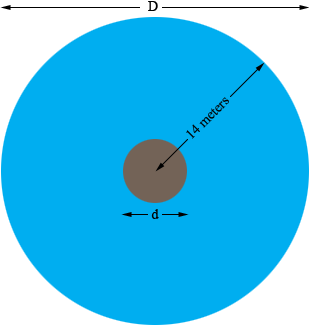SEARCH HOMEMath Central Quandaries & QueriesQuestion from kris, a parent: A fountain has a radius of 14 meters to its outer edge. There is an inner ring in the center of the fountain, where a statue of Sir Isaac Newton stands, that does not contain water. The inner ring has a diameter that is 6m less than the diameter of the outer ring of the fountain. What is the circumference of the inner ring? What is the area that is covered by water in the fountain?Hi Kris,

Suppose the diameter of the fountain is $D$ meters and the diameter of the inner ring is $d$ meters.What is $D?$

$d$ is 6 meters less than $D.$ What is $d?$

What is the radius of the inner ring?

What is the circumference of the inner ring?

What is the area of a circle with radius 14 meters?

What is the area of the inner circle?

PennyMath Central is supported by the University of Regina and The Pacific Institute for the Mathematical Sciences.